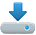1. The statistical approach to the verification of the beam structures buckling

Britvin E.I., Site Selenasys.com, 2012.

Abstract: According to the current understanding of the loss of stability nature of rod systems, the reason that the rod elements of structures lose stability under the longitudinal forces smaller than Euler's theory predicts, is the presence of small initial random bends and eccentricities when attaching rods. Therefore, even if the rod is centrally compressed, bending moments appear in it and if the fiber stresses at some point exceed the yield strength of the material, the process of buckling begins to develop at a catastrophic rate. In this paper, we propose a statistical approach to verifying the buckling of rod structures - several random samples of irregularities are superimposed on the rod elements, and several calculations are performed for a given load by a P-Delta analysis. After that, by statistical processing of the results for each rod, a conclusion is made about the probability of exceeding the yield strength of the material by the fiber stress.

2.Analysis of frame structures formed of thin-walled bar elements

Britvin E.I., Structural Mechanics and Analysis of Constructions, 2016. № 4. P. 43-54.

Abstract: Within the bounds of classic thin-walled bars theory the method of spatial frame structures formed from thin-walled bars analysis is proposed. The method taking into account the warping inequality of attached to node sections. The node is considered as the one-point finite element with n degrees of warping freedoms according to number of attached elements. Give the method for a node stiffness matrix constructing. The method based on a combination of finite element model analysis with followed by its "compression" thought the analytical development. It is shown that the elements of the node structure act as elastic warping external constraints imposed on the node. Give a method of finding the stiffness values of these constraints. The effectiveness of the method is demonstrated.

3.Influence of shear strain onto bending and torsion of thin-walled bars

Britvin E.I., Structural Mechanics and Analysis of Constructions, 2016. № 5. P. 59-65.

Abstract: At the concrete example illustrated how much importance it is to take into account influence of shear deformation when formation of the stiffness matrix of thin-walled bars. Effect of warping "without torsion" appearance is described for asymmetrical cross sections.

4.Equations of thin-walled bars deformation of open cross-sections with accounting for shear strains

Britvin E.I., Structural Mechanics and Analysis of Constructions, 2017. № 2. P. 44-51.

Abstract:It is generally assumed that the shear strain has little effect on the stress state of the rods. Noticeable this effect is only in sufficiently short rods. At the same time, as has been shown in a number of studies, this influence can be very significant for thin-walled rods already for rods of medium length. A problem of accounting for the effect of shear deformation becomes especially significant when you try to build a model of the unit formed by the connection of thin-walled rods , since an adequate description of the model requires the most exact correspondence of the analytical and finite element models of the rod. In this paper, we derive the deformation equations for thin-walled rods of an open profile with consideration of the shear strain. Expressions for warping and bimoment are given taking into account the shear deformation. Deduce the formulas for calculating of the shear coefficient for various sections. The effect of the appearance of warping in the cross-sections of asymmetric thin-walled rods is shown while the displacement or rotation of its ends without torsion. The conclusions of the theory tested by comparing with the results obtained in the finite element models.

5.Stiffness matrix of thin-walled bars with influence of shear strain formation

Britvin E.I., Structural Mechanics and Analysis of Constructions, 2017. № 1. P. 23-28.

Abstract:The stiffness matrix of thin-walled open section bars is constructed basis on the analytical solution of the complete system of equations of deformation of thin-walled section. The solution is given in view of a shear deformation of the lateral forces as well of the torque responsible for bending torsion.

Note:the article was mistakenly published earlier than article No. 4 (Equations of thin-walled bars deformation of open cross-sections with accounting for shear strains). The correct chronological sequence is restored in this list.

6.About torsion of the closed thin-walled sections.

Britvin E.I., Structural Mechanics and Analysis of Constructions, 2017. № 3. P. 36-45.

Abstract:Works on the problem of torsion of thin-walled rods of a closed section take their origin from the 30s-40s of the last century. Fundamental in this area are the works of A.A. Umansky. Mathematically, the theory of A.A.Umansky is very close to the shiftless theory of V.Z.Vlasov's thin-walled rods, but it allows one to obtain more or less reliable result for thin-walled rods of a closed section. Nevertheless, as experiments show, however the predictions of A.A.Umansky's theory are sometimes very far from reality. In this paper, we obtain the equations of torsion of thin-walled rods of a closed section taking into account three factors: shearing stresses of free torsion, warping and distortion of the section’s shape in its plane. An analytical solution of the basic equations is given. A comparison of of the theory predictions with the A.A.Umansky theory and with experimental data is made. Good agreement with experiment was obtained.

7.To the question on the determination of the buckling lengths of the rod elements of the frame space systems.

Britvin E.I., Structural Mechanics and Analysis of Constructions, 2019. № 3. P. 31-38.

Abstract:An efficient computational algorithm was constructed, which allows to find the buckling lengths of the rod elements of frame structures. A matrix of reaction from the side of the dropped part of the system is formed for each element and the problem of the eigenvalues of longitudinal bending equation of the rod is solved. When searching for eigenvalues, the stress state of the system is fixed. The solution is exact. High speed of algorithm is demonstrated.